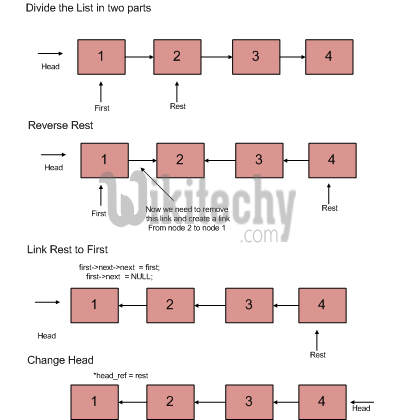# Python Algorithm – Write a function to reverse a linked list

Python Algorithm - Write a function to reverse a linked list - Linked List - Given pointer to the head node of a linked list, the task is to reverse

Examples:

```Input : Head of following linked list
1->2->3->4->NULL
Output : Linked list should be changed to,
4->3->2->1->NULL

1->2->3->4->5->NULL
Output : Linked list should be changed to,
5->4->3->2->1->NULL

Input : NULL
Output : NULL

Input  : 1->NULL
Output : 1->NULL```

Iterative Method
Iterate trough the linked list. In loop, change next to prev, prev to current and current to next

Python Programming:

``````# Python program to reverse a linked list
# Time Complexity : O(n)
# Space Complexity : O(1)

# Node class
class Node:

# Constructor to initialize the node object
def __init__(self, data):
self.data = data
self.next = None

def __init__(self):

# Function to reverse the linked list
def reverse(self):
prev = None
while(current is not None):
next = current.next
current.next = prev
prev = current
current = next

# Function to insert a new node at the beginning
def push(self, new_data):
new_node = Node(new_data)

def printList(self):
while(temp):
print temp.data,
temp = temp.next

# Driver program to test above functions
llist.push(20)
llist.push(4)
llist.push(15)
llist.push(85)

llist.printList()
llist.reverse()
llist.printList()

# This code is contributed by Nikhil Kumar Singh(nickzuck_007)``````
```Given linked list
85 15 4 20
20 4 15 85```

Time Complexity: O(n)
Space Complexity: O(1)

Recursive Method:

```   1) Divide the list in two parts - first node and rest of the linked list.
2) Call reverse for the rest of the linked list.C Programming:

``````void recursiveReverse(struct node** head_ref)
{
struct node* first;
struct node* rest;

/* empty list */
return;

/* suppose first = {1, 2, 3}, rest = {2, 3} */
rest  = first->next;

/* List has only one node */
if (rest == NULL)
return;

/* reverse the rest list and put the first element at the end */
recursiveReverse(&rest);
first->next->next  = first;

/* tricky step -- see the diagram */
first->next  = NULL;

/* fix the head pointer */
}``````

Time Complexity: O(n)
Space Complexity: O(1)

Python Programming:

``````# Simple and tail recursive Python program to

# Node class
class Node:

# Constructor to initialize the node object
def __init__(self, data):
self.data = data
self.next = None

def __init__(self):

def reverseUtil(self, curr, prev):

# If last node mark it head
if curr.next is None :

# Update next to prev node
curr.next = prev
return

# Save curr.next node for recursive call
next = curr.next

# And update next
curr.next = prev

self.reverseUtil(next, curr)

# This function mainly calls reverseUtil()
# with previous as None
def reverse(self):
return

# Function to insert a new node at the beginning
def push(self, new_data):
new_node = Node(new_data)

def printList(self):
while(temp):
print temp.data,
temp = temp.next

# Driver program
llist.push(8)
llist.push(7)
llist.push(6)
llist.push(5)
llist.push(4)
llist.push(3)
llist.push(2)
llist.push(1)

llist.printList()

llist.reverse()

llist.printList()

# This code is contributed by Nikhil Kumar Singh(nickzuck_007)``````

Output:

```Given linked list
1 2 3 4 5 6 7 8

8 7 6 5 4 3 2 1```
READ  Python Programming - Matrix Chain Multiplication